International
Tables for
Crystallography
Volume D
Physical properties of crystals
Edited by A. Authier

International Tables for Crystallography (2006). Vol. D, ch. 1.1, p. 8

## Section 1.1.3.4. Tensor nature of physical quantities

A. Authiera*

aInstitut de Minéralogie et de la Physique des Milieux Condensés, Bâtiment 7, 140 rue de Lourmel, 75015 Paris, France
Correspondence e-mail: aauthier@wanadoo.fr

#### 1.1.3.4. Tensor nature of physical quantities

| top | pdf |

Let us first consider the dielectric constant. In the introduction, we remarked that for an isotropic medium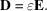If the medium is anisotropic, we have, for one of the components,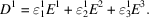This relation and the equivalent ones for the other components can also be written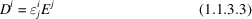using the Einstein convention.

The scalar product of D by an arbitrary vector x is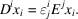The right-hand member of this relation is a bilinear form that is invariant under a change of basis. The set of nine quantities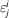constitutes therefore the set of components of a tensor of rank 2. Expression (1.1.3.3)is the contracted product ofby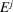.

A similar demonstration may be used to show the tensor nature of the various physical properties described in Section 1.1.1, whatever the rank of the tensor. Let us for instance consider the piezoelectric effect (see Section 1.1.4.4.3). The components of the electric polarization,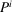, which appear in a medium submitted to a stress represented by the second-rank tensor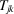are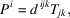where the tensor nature ofwill be shown in Section 1.3.2. If we take the contracted product of both sides of this equation by any vector of covariant components, we obtain a linear form on the left-hand side, and a trilinear form on the right-hand side, which shows that the coefficients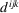are the components of a third-rank tensor. Let us now consider the piezo-optic (or photoelastic) effect (see Sections 1.1.4.10.5and 1.6.7). The components of the variation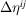of the dielectric impermeability due to an applied stress are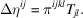In a similar fashion, consider the contracted product of both sides of this relation by two vectors of covariant componentsand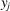, respectively. We obtain a bilinear form on the left-hand side, and a quadrilinear form on the right-hand side, showing that the coefficients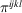are the components of a fourth-rank tensor.